# Hexagon - MO

The picture shows the ABCD square, the EFGD square and the HIJD rectangle. Points J and G lie on the side CD and is true |DJ| <|DG| and the points H and E lie on the DA side, with the |DH| < |DE|. We also know that |DJ| = |GC|. The hexagon ABCGFE has a perimeter 96 cm and hexagon EFGJIH having a perimeter 60 cm and a rectangle HIJD have perimeter 28 cm. Find the area of the hexagon EFGJIH.

Correct result:

S =  180 cm2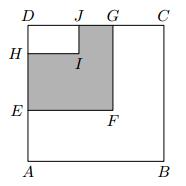We would be pleased if you find an error in the word problem, spelling mistakes, or inaccuracies and send it to us. Thank you!#### You need to know the following knowledge to solve this word math problem:

We encourage you to watch this tutorial video on this math problem:

## Next similar math problems:Convert magnitude of the angle α = 136°18'10" to radians:
• Angles 1It is true neighboring angles have not common arm?When Bedrich is as old as Adam today, Adam will be 14 years old. When Adam will be as old as Bedrich, Bedrich was two years old today. How old are Adam and Bedrich today?
• Three digits number 2Find the number of all three-digit positive integers that can be put together from digits 1,2,3,4 and which are subject to the same time has the following conditions: on one positions is one of the numbers 1,3,4, on the place of hundreds 4 or 2.
• SlopeFind the slope of the line: x=t and y=1+t.
• Isosceles trapezoid v3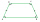In an isosceles trapezoid ABCD is the size of the angle β = 81° Determine size of angles α, γ and δ.
• Trapezium 2Trapezium has an area of 24 square cms. How many different trapeziums can be formed ?
• Square prismA square prism has a base with a length of 23 centimeters, what is the area in square centimeters of the base of the prism?
• Complementary angles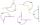Two angles are complementary. If one angle measures 10°, what is the measure of the second angle?
• Perimeter of squareThe square has a circumference 17cm. What is its area?
• MO 2016 Numerical axisCat's school use a special numerical axis. The distance between the numbers 1 and 2 is 1 cm, the distance between the numbers 2 and 3 is 3 cm, between the numbers 3 and 4 is 5 cm and so on, the distance between the next pair of natural numbers is always i
• 9-gon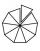Sum of interiol angles of 9-gon is:
• Draw it!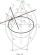Draw two lines c, d that c || d. On line c mark the points A, B. By point A lead perpendicular line to c. By point B lead perpendicular line to c.
• DecideThe rectangle is divided into seven fields. On each box is to write just one of the numbers 1, 2 and 3. Mirek argue that it can be done so that the sum of the two numbers written next to each other was always different. Zuzana (Susan) instead argue that i
• 6-gon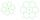Perimeter of regular hexagon is 113. Calculate its circumradius (radius of circumscribed circle).
• SidewalkThe city park is a circular bed of flowers with a diameter of 8 meters, around it the whole length is 1 meter wide sidewalk . What is the sidewalk area?
• Areaf of ST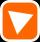It is given square DBLK with side |BL|=13. Calculate area of triangle DKU if vertex U lie on line LB.